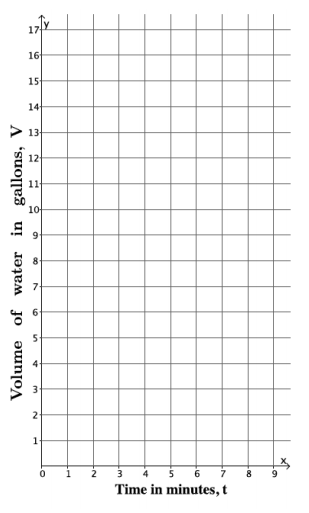# Linear Relationships

## Objective

Graph proportional relationships and interpret slope as the unit rate.

## Common Core Standards

### Core Standards

?

• 8.EE.B.5 — Graph proportional relationships, interpreting the unit rate as the slope of the graph. Compare two different proportional relationships represented in different ways. For example, compare a distance-time graph to a distance-time equation to determine which of two moving objects has greater speed.

?

• 6.EE.C.9

• 7.RP.A.2

## Criteria for Success

?

1. Draw graphs for proportional relationships in the coordinate plane.
2. Identify the slope of the graph and interpret it in context as the unit rate of the situation.

## Tips for Teachers

?

The following materials may be helpful for this lesson: graph paper.

### Remote Learning Guidance

If you need to adapt or shorten this lesson for remote learning, we suggest prioritizing Anchor Problem 1 (benefits from worked example). Find more guidance on adapting our math curriculum for remote learning here.

#### Fishtank Plus

Subscribe to Fishtank Plus to unlock access to additional resources for this lesson, including:

• Problem Set
• Student Handout Editor
• Google Classrom Integration
• Vocabulary Package

## Anchor Problems

?

### Problem 1

Water flows at a constant rate out of a faucet. Suppose the volume of water that comes out in three minutes is 10.5 gallons.1. Write a linear equation to represent the volume of water, $v$, that comes out of the faucet in $t$ minutes.
2. Find the volume of water out of the faucet after 0 minutes, 1 minute, and 4 minutes.
3. Graph the equation in the coordinate plane.
4. What is the slope of the graph? What does it mean as a unit rate?

#### Guiding Questions

Create a free account or sign in to access the Guiding Questions for this Anchor Problem.

#### References

EngageNY Mathematics Grade 8 Mathematics > Module 4 > Topic B > Lesson 11Example 2

Grade 8 Mathematics > Module 4 > Topic B > Lesson 11 of the New York State Common Core Mathematics Curriculum from EngageNY and Great Minds. © 2015 Great Minds. Licensed by EngageNY of the New York State Education Department under the CC BY-NC-SA 3.0 US license. Accessed Dec. 2, 2016, 5:15 p.m..

Modified by Fishtank Learning, Inc.

### Problem 2

Nia and Trey both had a sore throat so their mom told them to gargle with warm salt water.

• Nia mixed 1 teaspoon salt with 3 cups water.
• Trey mixed ${1\over 2}$ teaspoon salt with 1${{1\over2}}$cups of water.

Nia tasted Trey’s salt water. She said,
“I added more salt so I expected that mine would be more salty, but they taste the same.”

1. Explain why the salt water mixtures taste the same.
2. Find an equation that relates $s$, the number of teaspoons of salt, with $w$, the number of cups of water, for both of these mixtures.
3. Draw the graph of your equation from part (b).
4. Your graph in part (c) should be a line. Interpret the slope as a unit rate.

#### Guiding Questions

Create a free account or sign in to access the Guiding Questions for this Anchor Problem.

#### References

Illustrative Mathematics Sore Throats, Variation 2

Sore Throats, Variation 2, accessed on Feb. 23, 2018, 11:02 p.m., is licensed by Illustrative Mathematics under either the CC BY 4.0 or CC BY-NC-SA 4.0. For further information, contact Illustrative Mathematics.

## Problem Set

?With Fishtank Plus, you can download a complete problem set and answer key for this lesson. Download Sample

The following resources include problems and activities aligned to the objective of the lesson that can be used to create your own problem set.

## Target Task

?

At the grocery store, sliced turkey deli meat is on sale for \$11 for 2 pounds.

a.   What is the cost per pound of sliced turkey deli meat?

b.   Write an equation to represent the cost, $y$, of sliced turkey deli meat measured in $x$ pounds.

c.   Draw a graph of the situation.

d.   What is the meaning of the slope of the line that you drew?

#### Mastery Response

Create a free account or sign in to view Mastery Response